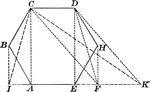### Hexagon Used to Construct Equivalent Triangle

Illustration of a how to construct a triangle equivalent to a given hexagon/polygon.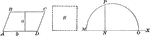### Parallelogram Used to Construct Equivalent Square

Illustration of a how to construct a square equivalent to a given parallelogram.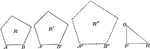### Pentagon Constructed to be Similar to Given Pentagons

Illustration of a how to construct a pentagon similar to two given pentagons.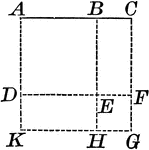### Square Constructed Upon the Sum of Two Straight Lines

Illustration of a square constructed upon the sum of two straight lines.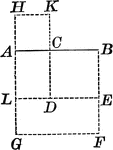### Square Constructed Upon Difference of Lines

Illustration of a square constructed upon the difference of two straight lines.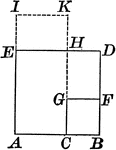### Square Constructed Upon Difference of Lines

Illustration of a square constructed upon the difference of two straight lines.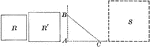### Square Constructed to be Equal to the Sum of Two Given Squares

Illustration of a how to construct a square equivalent to the sum of two given squares.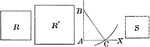### Square Constructed to be Equal to the Difference of Two Given Squares

Illustration of a how to construct a square equivalent to the difference of two given squares.